Updating search results...

# 22 Results

View
Selected filters:
• NC.M1.F-LE.5Conditional Remix & Share Permitted
CC BY-NC-SA
Rating
0.0 stars
Overview:

Students create models and understand the differences between linear and exponential models that are represented in different ways.

Subject:
Math 1
Material Type:
Lesson
Author:
EngageNY
02/09/2020Conditional Remix & Share Permitted
CC BY-NC-SA
Rating
0.0 stars
Overview:

Students apply knowledge of exponential functions and transformations of functions to a contextual situation.

Subject:
Math 1
Material Type:
Lesson
Author:
EngageNY
02/09/2020Conditional Remix & Share Permitted
CC BY-NC-SA
Rating
0.0 stars
Overview:

Students apply knowledge of exponential functions and transformations of functions to a contextual situation.

Subject:
Math 1
Material Type:
Lesson
Author:
EngageNY
02/09/2020Conditional Remix & Share Permitted
CC BY-NC-SA
Rating
0.0 stars
Overview:

This task explores the real world topic of building light rails. Throughout the implementation of this task the students will learn about the cost of building railways and how to implement them within a budget. This task explores such mathematical concepts of using coordinates to find the distance between points, using coordinates to build polygons and find the area and length of sides, and writing equations of parallel lines.

Subject:
Algebra
Geometry
Material Type:
Activity/Lab
Author:
Carrie Robledo
James O'Neal
03/26/2021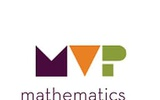Unrestricted Use
CC BY
Rating
0.0 stars
Overview:

The purpose of this task is to solidify and extend the idea that geometric sequences have
a constant ratio between consecutive terms to include sequences that are decreasing (0 < r < 1).
The common ratio in one geometric sequence is a whole number and in the other sequence it is a
percent. This task contains an opportunity to compare the growth of arithmetic and geometric
sequences. This task also provides practice in writing and using formulas for arithmetic sequences.

Subject:
Math 1
Material Type:
Activity/Lab
Author:
The Mathematics Vision Project
02/23/2020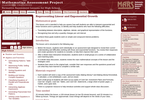Only Sharing Permitted
CC BY-NC-ND
Rating
4.0 stars
Overview:

This lesson unit is intended to help teachers assess how well students are able to interpret exponential and linear functions and in particular to identify and help students who have the following difficulties: translating between descriptive, algebraic and tabular data, and graphical representation of the functions; recognizing how, and why, a quantity changes per unit intervale; and to achieve these goals students work on simple and compound interest problems.

Subject:
Algebra
Geometry
Material Type:
Assessment
Lesson Plan
Provider:
Shell Center for Mathematical Education
Provider Set:
Mathematics Assessment Project (MAP)
06/24/2019Rating
4.0 stars
Overview:

Unit that compares the growth rates of linear, exponential and quadratic functions.

Subject:
Mathematics
Material Type:
Activity/Lab
Provider:
Weebly
02/12/2018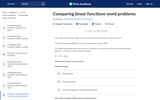Rating
0.0 stars
Overview:

In this Khan Academy activity, students will compare features of linear functions in word problems.

Subject:
Mathematics
Material Type:
Interactive
Provider:
09/06/2018Unrestricted Use
CC BY
Rating
0.0 stars
Overview:

This task is to solidify understanding that geometric sequences have a constant ratio
between consecutive terms. The task is designed to generate tables, graphs, and both recursive and
explicit formulas. The focus of the task should be to identify how the constant ratio shows up in
each of the representations.

Subject:
Math 1
Material Type:
Activity/Lab
Author:
The Mathematics Vision Project
02/23/2020Conditional Remix & Share Permitted
CC BY-NC-SA
Rating
0.0 stars
Overview:

The purpose of the task is to build fluency with the procedural work of linear and exponential functions. This task is designed to help students recognize the information given in a problem and use it efficiently. In the task, students will work with both linear and exponential functions given in tables, graphs, equations, and story contexts. They will construct various representations, with an emphasis on writing equations using various forms and using equations to graph the functions.

Subject:
Math 1
Material Type:
Lesson
Author:
Mathematics Vision Project
03/10/2020Unrestricted Use
CC BY
Rating
0.0 stars
Overview:

The purpose of this task is to compare the rates of growth of an exponential and a linear function.
The task provides an opportunity to look at the growth of an exponential and a linear function for
large values of x, showing that increasing exponential functions become much larger as x increases.
This task is a good opportunity to model functions using technological tools and to discuss how to
set appropriate viewing windows for functions. The task also leads to a discussion of whether this
particular situation should be modeled using discrete or continuous functions.

Subject:
Math 1
Material Type:
Activity/Lab
Author:
The Mathematics Vision Project
02/23/2020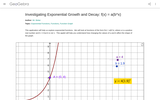Rating
4.0 stars
Overview:

Interactive geogebra activity all about geometric growth and decay.

Subject:
Mathematics
Material Type:
Interactive
Provider:
GeoGebra
05/30/2018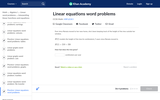Rating
0.0 stars
Overview:

In this Khan Academy activity, students will evaluate linear equations from words problems.

Subject:
Mathematics
Material Type:
Interactive
Provider:
09/12/2018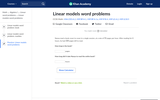Rating
0.0 stars
Overview:

Subject:
Mathematics
Material Type:
Interactive
Provider:
09/07/2018Unrestricted Use
CC BY
Rating
0.0 stars
Overview:

This is the first task of two that focus on understanding and using various notations for linear
functions. The task involves students in thinking about a context where students have selected the
index in two different ways, thus getting two different, but equivalent equations. The idea is
extended so that students can see the relationship expressed in point/slope form of the equation of
the line.

Subject:
Math 1
Material Type:
Activity/Lab
Author:
The Mathematics Vision Project
02/23/2020Conditional Remix & Share Permitted
CC BY-NC-SA
Rating
0.0 stars
Overview:

Students are given 4-5 coordinate points, that will represent the sheep.  First, they are tasked with creating a fence around the sheep. The students will use the endpoints of each piece of fence to compute the slope, y-intercept, domain and equation of the line. They will then code the rover to draw their lines. Finally, the students can calculate the area/perimeter of their fence and calculate the cost to build the fence. The students will then be challenged to minimize the area, perimeter and cost of building their corral, recalculate the equations of their lines, stating their new slope, y-intercept, and domain.

Subject:
Mathematics
Material Type:
Activity/Lab
Author:
William Allred
Carrie Robledo
05/18/2021Conditional Remix & Share Permitted
CC BY-NC-SA
Rating
0.0 stars
Overview:

Students are given 5 random coordinate points and must create a path of linear equations to navigate the rover around each point. Teachers can have students calculate the slope, y-intercept, domain and range of the lines. Students will calculate the distance of each line and find the total distance the rover travelled. Students will then be challenged to minimize the distance the rover travelled. Teachers can extend this to include angles and distance, where the students calculate the angle the rover should turn and then the distance it should drive to go around each point.

Subject:
Math 1
Material Type:
Activity/Lab
Author:
William Allred
Carrie Robledo
05/18/2021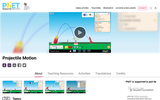Unrestricted Use
CC BY
Rating
0.0 stars
Overview:

Sample Learning Goals
Determine how each parameter (initial height, initial angle, initial speed, mass, diameter, and altitude) affects the trajectory of an object, with and without air resistance.
Predict how varying the initial conditions will affect a projectile’s path, and provide an explanation for the prediction.
Estimate where an object will land, given its initial conditions.
Determine that the x and y motion of a projectile are independent.
Investigate the variables that affect the drag force.
Describe the the effect that the drag force has on the velocity and acceleration.
Discuss projectile motion using common vocabulary (such as: launch angle, initial speed, initial height, range, time).

Subject:
Mathematics
Material Type:
Activity/Lab
Author:
PhET Interactive Simulations
10/05/2021Only Sharing Permitted
CC BY-NC-ND
Rating
0.0 stars
Overview:

Students use linear functions and systems of linear functions to help create a space suit.

Subject:
Math 1
Material Type:
Activity/Lab
Formative Assessment
Author:
NASA
11/27/2019Unrestricted Use
CC BY
Rating
0.0 stars
Overview:

This task is to solidify understanding that arithmetic sequences have a constant difference between
consecutive terms. The task is designed to generate tables, graphs, and both recursive and explicit
formulas. The focus of the task should be to identify how the constant difference shows up in each
of the representations and defines the functions as an arithmetic sequence.

Subject:
Math 1
Material Type:
Activity/Lab
Author:
The Mathematics Vision Project